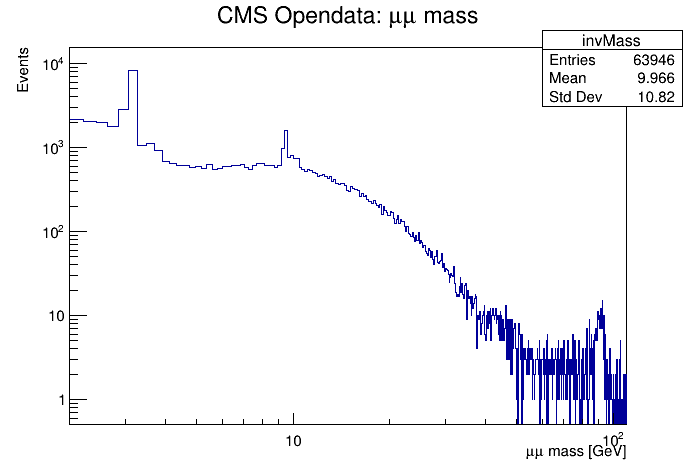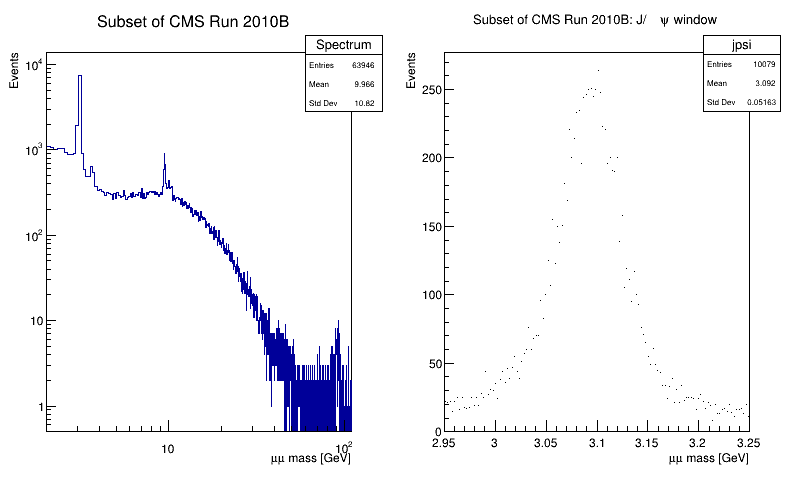# Df 0 1 4_ C S V Data Source¶

This tutorial illustrates how use the RDataFrame in combination with a RDataSource. In this case we use a TCsvDS. This data source allows to read a CSV file from a RDataFrame. As a result of running this tutorial, we will produce plots of the dimuon spectrum starting from a subset of the CMS collision events of Run2010B. Dataset Reference: McCauley, T. (2014). Dimuon event information derived from the Run2010B public Mu dataset. CERN Open Data Portal. DOI: 10.7483/OPENDATA.CMS.CB8H.MFFA.

Author: Enric Tejedor
This notebook tutorial was automatically generated with ROOTBOOK-izer from the macro found in the ROOT repository on Monday, July 06, 2020 at 11:35 AM.

In :
import ROOT
import os

Welcome to JupyROOT 6.23/01


Let's first create a RDF that will read from the CSV file. The types of the columns will be automatically inferred.

In :
fileNameUrl = "http://root.cern.ch/files/tutorials/df014_CsvDataSource_MuRun2010B.csv"
fileName = "df014_CsvDataSource_MuRun2010B_py.csv"
if not os.path.isfile(fileName):
ROOT.TFile.Cp(fileNameUrl, fileName)

MakeCsvDataFrame = ROOT.RDF.MakeCsvDataFrame
df = MakeCsvDataFrame(fileName)

[TFile::Cp] Total 14.46 MB	|====================| 100.00 % [61.4 MB/s]


Now we will apply a first filter based on two columns of the CSV, and we will define a new column that will contain the invariant mass. Note how the new invariant mass column is defined from several other columns that already existed in the CSV file.

In :
filteredEvents = df.Filter("Q1 * Q2 == -1") \
.Define("m", "sqrt(pow(E1 + E2, 2) - (pow(px1 + px2, 2) + pow(py1 + py2, 2) + pow(pz1 + pz2, 2)))")


Next we create a histogram to hold the invariant mass values and we draw it.

In :
invMass = filteredEvents.Histo1D(("invMass", "CMS Opendata: #mu#mu mass;#mu#mu mass [GeV];Events", 512, 2, 110), "m")

c = ROOT.TCanvas()
c.SetLogx()
c.SetLogy()
invMass.Draw()


We will now produce a plot also for the J/Psi particle. We will plot on the same canvas the full spectrum and the zoom in the J/psi particle. First we will create the full spectrum histogram from the invariant mass column, using a different histogram model than before.

In :
fullSpectrum = filteredEvents.Histo1D(("Spectrum", "Subset of CMS Run 2010B;#mu#mu mass [GeV];Events", 1024, 2, 110), "m")


Next we will create the histogram for the J/psi particle, applying first the corresponding cut.

In :
jpsiLow = 2.95
jpsiHigh = 3.25
jpsiCut = 'm < %s && m > %s' % (jpsiHigh, jpsiLow)
jpsi = filteredEvents.Filter(jpsiCut) \
.Histo1D(("jpsi", "Subset of CMS Run 2010B: J/#psi window;#mu#mu mass [GeV];Events", 128, jpsiLow, jpsiHigh), "m")


Finally we draw the two histograms side by side.

In :
dualCanvas = ROOT.TCanvas("DualCanvas", "DualCanvas", 800, 512)
dualCanvas.Divide(2, 1)

from ROOT import gROOT